# Coaching Materials in Acoustics Part 2 for ECE Board Exam

(Last Updated On: December 8, 2017)This is the Coaching materials in Acoustics Part 2 as one topic in ECE Board Exam taken from various sources including but not limited to past Board Examination Questions in Electronic System and Technologies (EST), Communications Books, Journals and other Communications References. This particular Coaching Notes in Communications Engineering has random Questions and Answers in random topics. Make sure to familiarize this review notes to increase the chance of passing the ECE Board Exam.

### Acoustics Coaching Materials Part 2

1. If the distance between the listener and the source is tripled, intensity is reduced to

1/9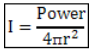2. Decreasing distance by ½, intensity increases

4 times *

3. How much is the reduction of sound intensity level (SIL) every time we double the distance?

6dB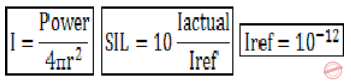4. A loudspeaker radiates 100mW, what is the sound intensity level (SIL) at a distance 1 m from the source?

99dB

5. Doubling the pressure level will result in a _____ increase in SPL.

6dB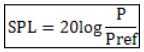6. What is the increase in sound intensity level (SIL) if the intensity if doubled?

3dB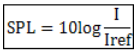7. What is the SPL for a sound wave with 1 Pa rms pressure amplitude?

94dB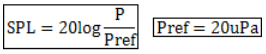8. SPL can also be calculated using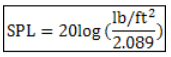9. Increase in SPL in dB, if pressure is increased 4 times,

12dB

10. A sound pressure (RMS)of 125 ubars is equivalent to what sound pressure level?

116dB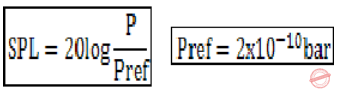11. The sound pressure level of 0 dB is equivalent to how many ubars?

2 x 10-4 or 0.0002

12. A loudspeaker has an effective area of 0.05 m^2 and radiates 20 mW. Calculate the sound intensity at the loudspeaker.

0.4W/m^2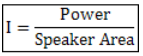13. Calculate the sound intensity level of the loudspeaker having a sound intensity of 0.4 W/m^2?

116dB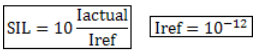14. If a sound source radiates 1 watt, what is its sound power level (SWL)?

120dB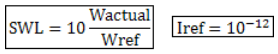15. Calculate the acoustic impedance if the sound pressure is 20uPa and acoustic intensity is 10-12 W/m2 .

400 kg/m2s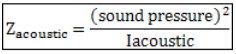16. What are the three basic characteristics of sound?

Pitch, intensity and quality

17. The sound characteristics related to a sonic time pattern is known as

rhythm

18. The two main characteristics of sound waves.

Pitch and loudness

19. A unit of loudness for an individual listener is

Sone

20. The unit loudness level of a tone compared to the intensity of 1000-Hz tone. It is the apparent change in loudness discerned by a listener.

Phon

21. A pure tone of loudness 40 phons produces a loudness sensation of

l sone

22. By definition, a pure tone 1000-Hz of loudness l sone produces a pitch of

1000 mels

23. In measuring loudness, using 1000-Hz sinewave as a reference, l sone is agreed to be at what level, above the listener’s threshold of hearing?

40dB

24. As defined by ANSI, _____ is that attribute of auditory sensation in terms of which sounds may be ordered on a scale extending from low to high.

Pitch

25. The unit of pitch is the

Mel

26. Pitch of sound is dependent on

sound frequency, sound waveform, and sound pressure.

27. The apparent change in frequency or pitch because of motion is explained by what effect?

Doppler effect

28. _____ surfaces must be avoided since they result in undesirable focusing of sound.

Concave

29. What term describes sounds capable of being heard by the human ear?

Sonics

30. A sound is considered as having a pitch if

it has a periodic acoustic pressure variation with time

31. Pitch perception theory which relates directly to the frequency analysis carried out by the basilar membrane in which different frequency components of the input sound stimulate different positions, or places on the membrane,

place theory

32. Pitch perception theory that is based on the fact that the waveform of a sound with a strong musical pitch is periodic. This theory relies on the timing of neural firings generated in the organ of Corti which occurs in response to vibrations of vasilar membrane.

Temporal theory

33. If a note has a fundamental frequency, fz =100 Hz, what is its 5th harmonic?

500Hz.

34. If a note has a fundamental frequency, fz =100 Hz, what is its 5th overtone?

600Hz.

35. The separation in pitch of frequency between two sounds.

Interval

36. The ratio of two sound frequencies separated by an interval.

Frequency interval

37. Interval between two tones sounded simultaneously.

Harmonic interval

38. Interval between two tones sounded successively.

Melodic interval

39. The interval between any two frequencies that have a ratio of 2 to 1.

Octave

40. 11th octave, above 1kHz.

2048Hz

41. Frequency 10 octaves above 30Hz.

30.72kHz

42. Octaves of frequency range 1 to 8 MHz.

3

43. In acoustics, if the low frequency edge of a band is 10Hz, what is the high frequency edge of the band 10 octaves wide?

10240Hz.

44. Sound wave deformation during reproduction of amplification.

Distortion

45. A means of correcting distortion

Equalization

Rate this: